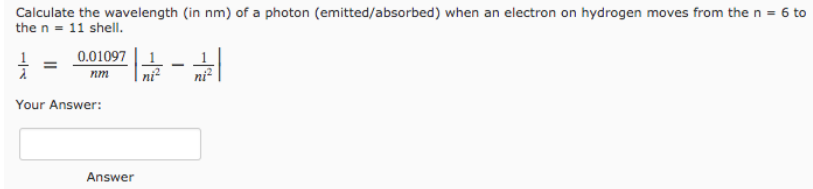# Problem: Calculate the wavelength (in nm) of a photon (emitted/absorbed) when an electron on hydrogen moves from the n = 6 to the n = 11 shell.

###### FREE Expert Solution###### Problem Details

Calculate the wavelength (in nm) of a photon (emitted/absorbed) when an electron on hydrogen moves from the n = 6 to the n = 11 shell.